# What are Different Types of Fluid Flow – Complete Explanation

## Introduction to Fluid

The fluid is a matter that tends to deform continuously under Shear Stress. It consists of both liquid and gases. All the matters on earth can be classified based on their behavior into two categories, i.e., Solid and Fluid. The substance that tends to flow under certain given conditions is called fluid. It becomes essential to understand different types of Fluid and the difference between fluid and solid for better understanding and visualization of types of fluid flow. In this article, we will be discussing types of fluid and the difference between solid and fluid in brief and then move onto types of Fluid flow.

## Types of Fluids

Fluids are classified based on their behavior under Shear stress. The Behaviour is analyzed with the help of Quantity called Viscosity and density of Fluid. Viscosity is like frictional force present in Solid Particles, which resist the Motion of Solid. It resists the fluid flow and induces a relative motion between the subsequent fluid layers.

To Classify and have a better understanding of classification, Consider the Following Equation: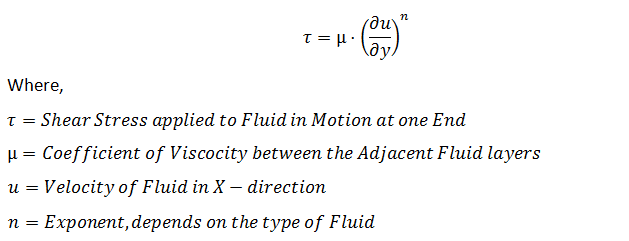Note: Above Equation represents the fluid flowing in One direction (X-direction), and flow in all Other Directions is Zero.

We can Classify Fluid into 6 Different types, which are discussed as follows: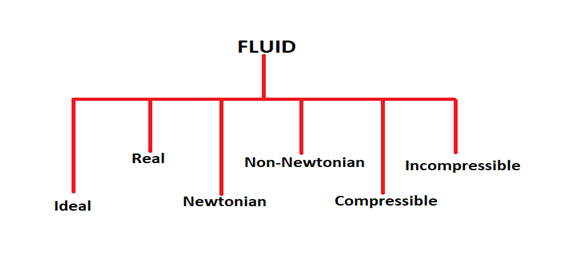### 1. Ideal Fluid:

In this type of Fluid, Viscosity is considered to be Zero, and Density is Constant Everywhere. It means that there is no relative motion between the layers of Fluid in Fluid flow and all the layers move with the same velocity. Ideal Fluid is an assumption, and they are not present in reality. These assumptions are made to analyze the behavior of some fluid at given conditions.

In Short, we can say that,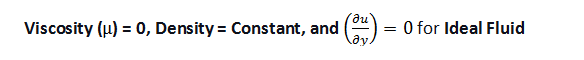### 2. Real Fluid:

In this type of Fluid, Viscosity is not zero, and Density varies everywhere in the Fluid. It means that there is relative motion between the layers of fluid in fluid flow. Real Fluid is the behavior possessed by Fluid in reality but is often ignored to make the analysis simpler. In Real Fluid, we don’t have any fixed formula for the variation of Density and fixed value for Fluid’s Viscosity. All the Fluids are real Fluid in Nature.

In Short, we can say that,### 3. Newtonian Fluid:

In real fluid, we don’t have the exact formula for calculating Density, and we don’t know the Viscosity of fluid. Newtonian Fluid is that fluid with a defined value of Viscosity, and the value of Exponent (n) is 1. The Equation for all Newtonian fluid can be written as follows:

Newtonian Fluid can have Constant and variable Density, but the Variation of Density

with respect to time and space will be known to us.

In short, we can say that,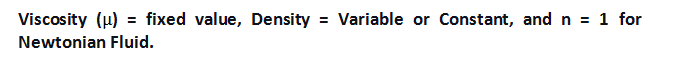### 4. Non-Newtonian Fluid:

In this type of Fluid, Viscosity is not zero and is defined accurately. Density can vary or remain constant with respect to Time and Space. The main difference arises with the value of Exponent ‘n’, which is not equal to 1 and depends on the type of Non-Newtonian fluid. The Equation for all Non-Newtonian Fluid can be written as follows: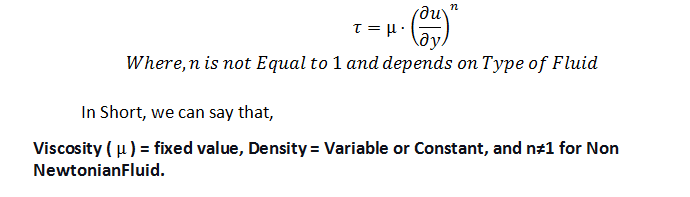### 5. Compressible Fluid:

The fluid is said to be compressible Fluid if density varies with time and space. We can’t say about the Viscosity in this case as it can either be Zero or non-Zero.

In short, we can say that,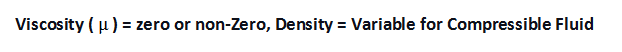### 6. Incompressible Fluid:

The fluid is said to be Incompressible if the density of Fluid doesn’t vary with time and space. We can’t say about the Viscosity in this case as it can either be Zero or non-Zero.

In short, we can say that,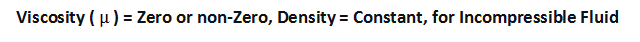The below Classification list the Property of different fluids on the basis of their Viscosity and density:

 S.no. Type of Fluid Viscosity Density 1 Ideal Fluid Zero Constant 2 Real Fluid Non-Zero Variable 3 Newtonian Fluid Non-Zero and have definite formula Can be either Constant or variable 4 Non-Newtonian Fluid Non-Zero and formula depends on type of Non-Newtonian fluid Can be either constant or Variable 5 Compressible Fluid Zero/Non-Zero Variable 6 Incompressible Fluid Zero/Non-Zero Constant

## Solid vs Fluid: Differences Between Them

Solid and fluid differ in their behavior and don’t follow the rules of Physics in the same manner. Because of their difference in properties, we have Solid Mechanics for Solid and Fluid Mechanics for Fluid. We can note many differences between them as we closely observe their Behaviour under various conditions. To group their Behaviour into a more straightforward manner, we use the property called Shear stress. It defines the flow property of fluid and how its behaviour is different from solids. Solids tend to bend and deform under the action of Shear stress. Hence, their shear stress varies linearly with bending or deformation. Fluid tends to Deform continuously under the action of Shear stress, and the variation of Shear stress is not Linear with Deformation. This is the main difference between Solid and fluid.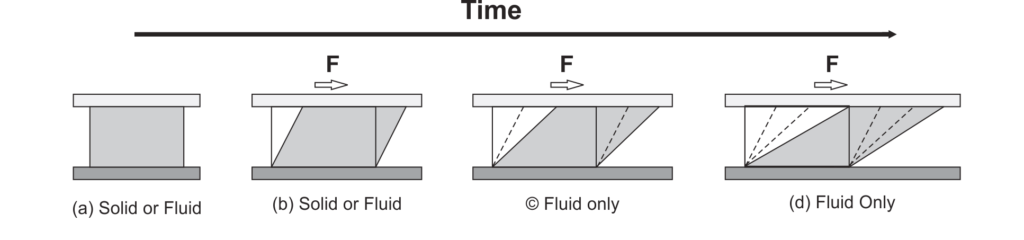Fig: Shear Stress Behaviour

## Driving Force for Fluid Flow

Flow Characteristics of Fluid lead to various Phenomena such as Cyclone, Change in Weathers, Cooling of Internal Combustion Engine, and many more things. The question arises, what is the reason for the flow of Fluid? And can we predict the Fluid flow behavior at every condition? Fluid Flows from One Point to another because of the Pressure difference between the two Points. The Natural Flow of Fluid Occurs from High-pressure to Low-Pressure to equalize the pressure difference at these two points. Flow from Low-Pressure to High-pressure region can be achieved with External Driving force, such as Pump, Etc. Fluid flow Patterns can be classified but can’t be accurately predicted at an Instant of time. All the Prediction for the Fluid flow behavior is made with the use of Softwares that use Numerical Techniques to Approximate the behavior of flow at a given instant.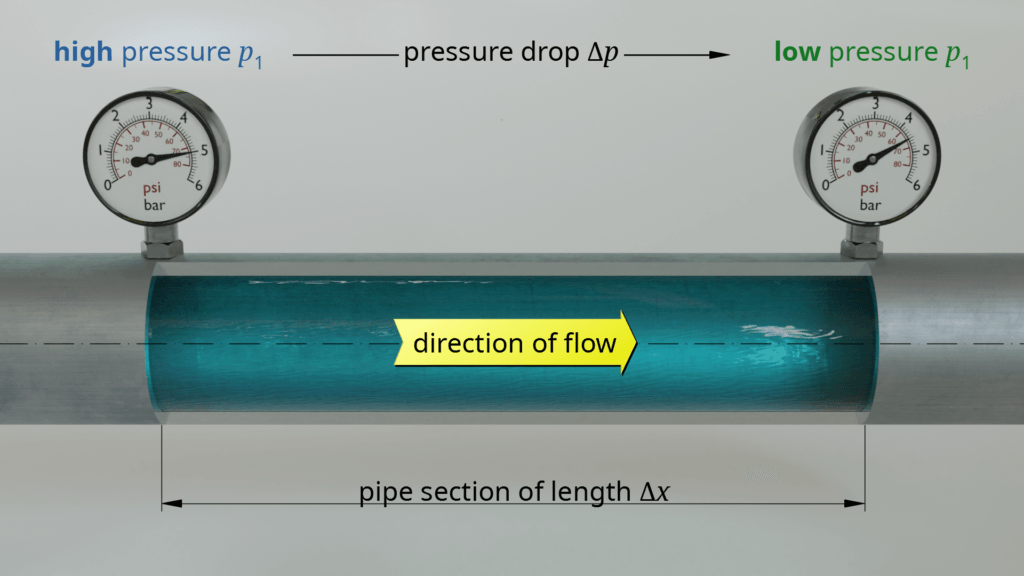Image Source

To Start Our Study for Fluid Flow, let us first learn about different Parameters in fluid flow. These Parameters are Timelines, Pathlines, Streaklines, and Streamlines.

### Timeline:

If we mark adjacent fluid particles’ flowing at a given instant of time, it forms a timeline. For Example, to demonstrate fluid particle behavior under the action of constant shear stress, the timeline was Introduced to give the deformation of Fluid at each Instant of time. Hence, In the timeline, Each and every Particle of Fluid is traced at a given Instant of time.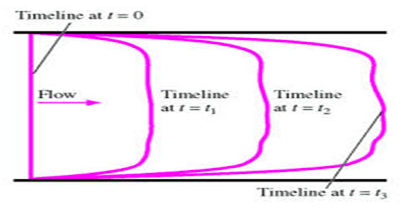Fig.4: Timeline

### Pathline:

If we trace the path of a fluid particle for some time, it forms Pathline. For Example, take dye and smoke, and take a long exposure photograph of its subsequent motion. The path traced by the Particle is Pathline. Here, we consider a source Particle of fluid and Observe its Path for a given Time. The Path traced by the Particles during that Time is the Pathline of the Particle.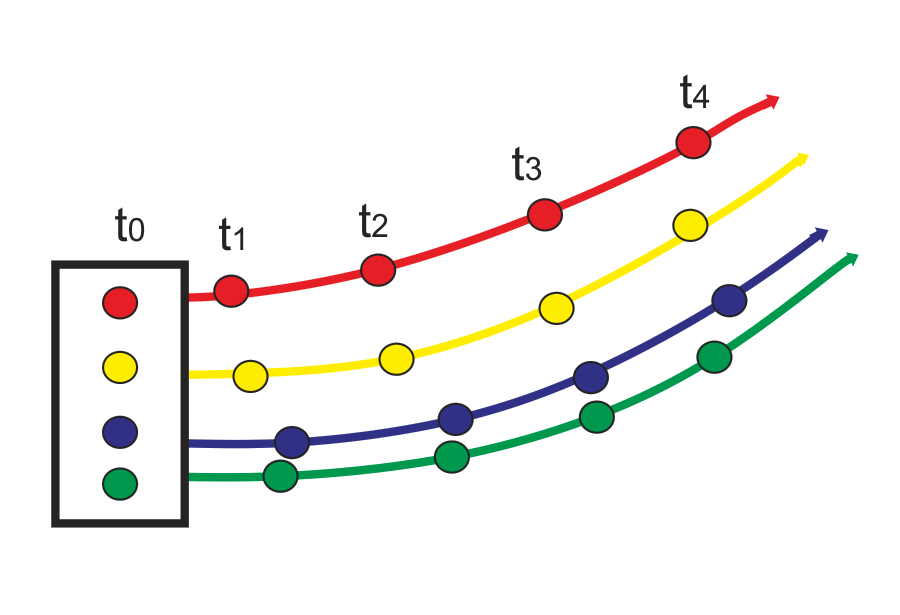Fig.: Pathline

### Streakline:

If we mark the Fluid particle Path at a given location for some time, it forms Streakline. During Wind tunnel aerodynamic testing of Automobile, smoke is released towards the car for aerodynamic force and Drag evaluation. The Path traced by the smoke over the car is Streakline. Here, we consider the flow of subsequent layers, observe their Position at a given Instant of time and trace the Position to form Streakline.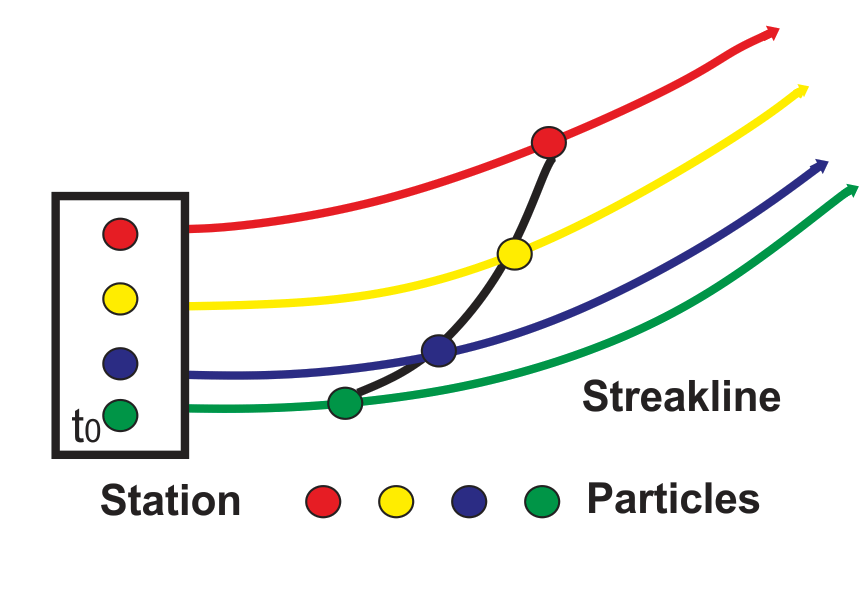Fig: Streakline

### Streamline:

It is the Path drawn for a fluid Particle so that tangent to it gives the direction of the velocity of fluid particles at the point. Since they are the tangent to flow, there can be no flow along the streamline. They are used in Computer Simulation for Flow Visualization, where Streamlines are drawn to represent velocity field traced by the Fluid Particles.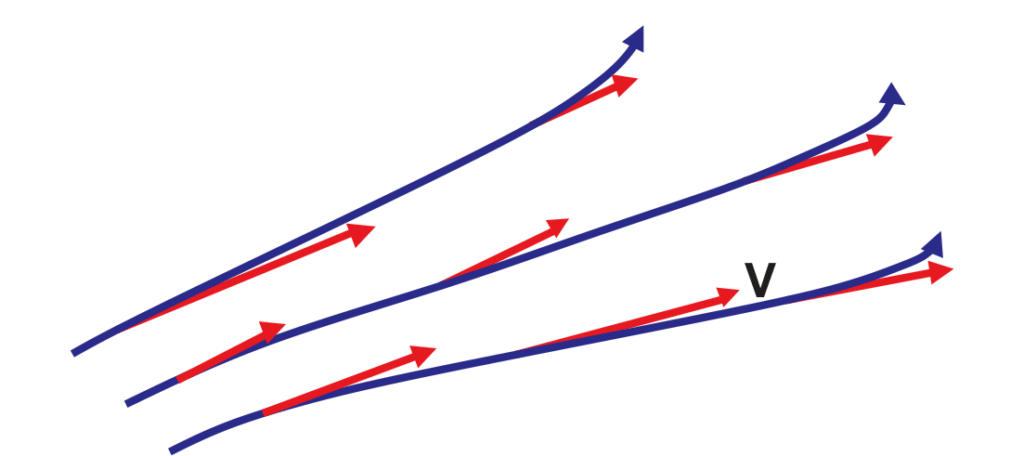Fig.: Streamline

## Types of Fluid Flow

Fluid flow can be classified into the following types:

1. Uniform and non-Uniform flow
3. Rotational and Irrotational Flow
4. Compressible and Incompressible Flow
5. Viscous and non-Viscous Flow
6. External and Internal Flow
7. Laminar and Turbulent flow
8. 1D, 2D, and 3D Flow

Let us Study about them one by one:

### 1. Uniform and non-Uniform Flow

Fluid flow is said to be Uniform if the Velocity of fluid does not change with Space. Hence, in this types of fluid flow, Velocity is only dependent on Time and not on X, Y, Z Coordinates of fluid Particle.

Fluid flow is said to non-Uniform if the Velocity of fluid changes with Space. Hence, in this type of flow, Velocity is the Function of Time and X, Y, Z Coordinates of Fluid particles. For Example, As shown in the figure, the Velocity of flow is constant when the cross-section area is not changing, but as the area of cross-section changes, the Velocity varies as the fluid moves into the section. The flow becomes non-Unifrom in nature.

The flow of fluid through a pipe with a uniform cross-section is called uniform flow and if the fluid flow is through a pipe with no uniform ( or tapered ) cross-section is called non-uniform flow

Fluid Flow is said to be Steady if Fluid Properties such as velocity and Pressure don’t vary with time. Hence, in this flow, Fluid Properties are only dependent on the X, Y, Z Coordinates of a fluid particle. For this type of flow, streaklines, streamlines, and pathlines are identical.

Fluid flow is Unsteady if fluid properties such as velocity and Pressure vary with time. Hence, in this flow, Fluid properties are dependent on time and X, Y, Z Coordinates of a fluid particle. For this type of flow, streaklines, streamlines, and pathlines are not identical.

In the Given figure, the first expression represents Steady flow, while the second expression is for Unsteady flow. A constant Discharge through Pipe will be a steady flow, while Variable discharge through the pipe will be Unsteady flow.

### 3. Rotational and Irrotational Flow

If the Fluid particles rotate about their axis while moving in the streamline, it is called Rotational flow.
If the fluid particles move in a streamline and don’t rotate about their axis, it is called Irrotational flow.
We can identify this type of flow by calculating the flow’s vorticity, which depends on the velocity of flow. If Vorticity is Zero, fluid flow is Irrotational; otherwise, it is rotational flow.

### 4. Compressible and Incompressible Flow

In Compressible flow, the density of fluid changes with time and space. While, In Incompressible flow, the density of fluid remains constant. This flow finds its Application in Brake Fluid. In the Braking System, Brake Fluid transfers the Pressure created by the foot to wheels for Breaking. If Fluid is Incompressible, it will transfer the Exact Pressure applied by the foot to the wheels for effective braking. If the fluid is Compressible, the Pressure transferred to the Wheel will be less than applied. It may even be zero. Hence, brake fluid should be Incompressible in Nature.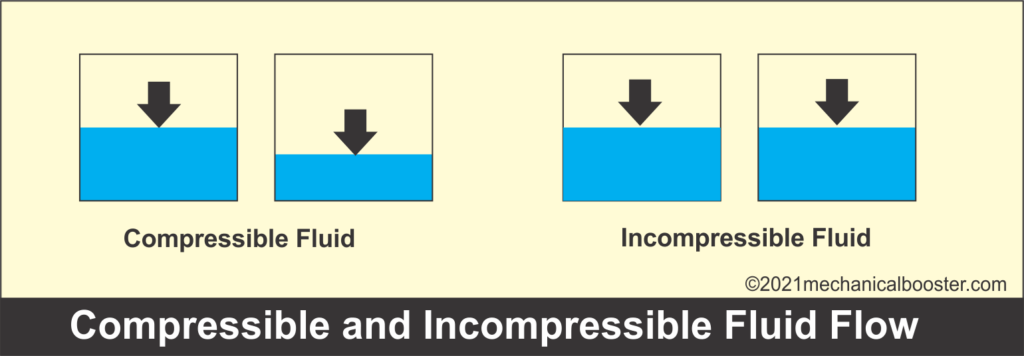We can quickly identify these types of fluid flow with the help of Mach Number. It is defined as:

Ma= V/Vs

• Vs= Velocity of Sound in fluid
• V= Velocity of fluid
 0<=Ma<0.33 Incompressible flow Ma>0.33 Compressible flow

### 5. Viscous and Non-Viscous Flow:

In Viscous flow, Fluid Particles experience viscosity between the Subsequent layers, and Hence, Relative motion occurs between the layer of fluid particles. In Non-Viscous Flow, Fluid Particles don’t experience any viscosity between the Subsequent layers, and Hence, there is no relative Motion between the Fluid Particles.

### 6. External and Internal Flow: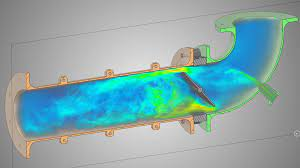Internal Fluid Flow

The Presence of the wall determines this type of flow Pattern. Flow Completely Bounded by a solid body is called Internal Flow or Duct Flow.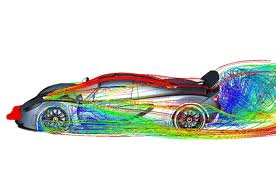If any Solid-body does not bound the Flow, it is called External Flow. For Example, Flow over an Automobile is called External Flow, which is visualized with the help of Softwares and Wind tunnel test. Flow inside a circular Pipe is Internal Flow and can be easily visualized with the help of Softwares and simple laboratory Experiments.

### 7. Laminar and Turbulent Flow

In Laminar Flow, the fluid particles move at different layers and don’t mix macroscopically. In this type of fluid Flow, we can predict the Pattern of Flow at a given instant of time. All the Subsequent layers are parallel to each Other in the Flow.

In Turbulent Flow, the fluid particles Intermix, and Flow become random. The pattern of Flow can’t be predicted accurately at a given instant of time in this type of Flow. Eddies formation takes place, which leads to a large amount of energy loss.

Reynolds number is used to predict Flow, i.e., Whether it is turbulent and Laminar. The formula is given by:

Re= V*L/µ

Where,

• Re = Reynolds number
• V= Velocity of fluid
• L= Characteristics length of Object where flow is taking place
• µ= Coefficient of Viscosity

For Internal Flow,

 0<=Re<=2000 Laminar Flow 2000

For External Flow,

 0<=Re<=100000 Laminar Flow 100000

### 8. 1-D, 2-D, and 3-D Fluid flow:

In the 1-D type of Fluid flow, Fluid parameters such as velocity are the function of time and One spatial Coordinate only.

In the 2-D types of Fluid flow, Fluid parameters such as velocity are the function of time and two spatial Coordinates.

In the 3-D Fluid flow, Fluid parameters such as velocity are the function of time and all three Spatial Coordinates.

 1-D fluid Flow u= f(x,t), v=0, and w=0 2-D fluid Flow u= f(x,y,t), v=g(x,y,t), w=0 3-D fluid Flow u= f(x,y,z,t), v=g(x,y,z,t), w=h(x,y,z,t)

FAQs

Q.1. What is the difference between Compressible Fluid and Compressible Flow?

Answer- Compressible Fluid talks about Fluid and its density variation, while compressible flow only talks about Fluid in Motion and the density variation of Fluid in Motion. Fluid can have a constant density during static conditions and variable density during dynamic condition. Mach Number determines whether the flow is compressible or not. It does not decide the fluid characteristics.

Q.2. Which type of Fluid Flow is considered in the transition regime?

Answer- It depends upon the Situation and on your Computer Condition. If you have a value near to turbulent in transition regime and have an excellent Computer for Simulation, go for turbulent. If not, then go for Laminar.

Q.3. Is Flow of air passed through the car is External and Internal Flow

Answer- If we analyze the aerodynamic drag of a car, it is external Flow. There is some amount of air that goes inside the car. Since it is bounded by Solid, Hence, can be considered as Internal Flow.

Q.4. What is the name of Law that is applied in the Application of Brake fluid?

Answer- The name of the law is Pascals law. It states that for an Incompressible fluid, Pressure is transferred equally in all the direction.

Q.5. Why is timeline is not mentioned in a steady and Unsteady flow?

Answer- There is no concept of timeline for Steady flow, since fluid parameters doesn’t depend on time.

Q.6. What is Pump?

Answer- Pump is an External agent that is used in movement of fluid, against their natural direction of flow. For Example, Pump is used in Steam Power plant for taking the water from Condenser to the Boiler at a height.

Q.7. What is Shear stress?

Answer- Shear stress is the stress developed by the force applied tangentially to the Object, on which the force is applied.